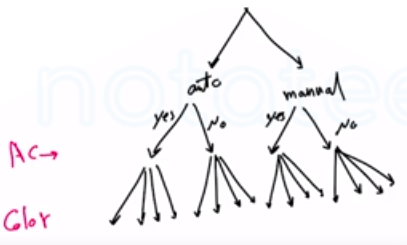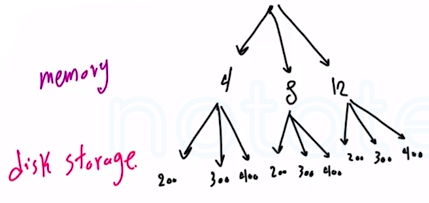Need Help?

Subscribe to Probability

###### \${selected_topic_name}
• Notes
• Comments & Questions

An order for an automobile can specify either an
automatic or a standard transmission, either with or without
air-conditioning, and any one of the four colors red, blue,
black or white. Describe the set of possible orders for this
experiment.S = {(aut, with AC, red), (aut, with AC, blue), ……}

$n(s)=16$

An order for a computer system can specify memory
of $4,8,$ or 12 gigabytes, and disk storage of $200,300,$ or 400
gigabytes. Describe the set of possible orders.$S=\{(4,200),(4,300),(4,400),(8,200) \ldots\}$

$n(s)=9$

Calls are repeatedly placed to a busy phone line until
a connect is achieved. describe the sample space

$b \rightarrow$ buy
$c \rightarrow$ connect

$S=\{c, b c, b b c, \dots\}$

$n(s) \rightarrow \infty$

No comments yet